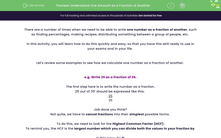# Understand One Amount as a Fraction of Another

In this worksheet, students will practise expressing amounts as fractions of each other and cancelling these fractions into their simplest forms, with and without units of measurements..Key stage:  KS 4

Year:  GCSE

GCSE Subjects:   Maths

GCSE Boards:   AQA, Eduqas, Pearson Edexcel, OCR,

Curriculum topic:   Ratio, Proportion and Rates of Change, Fractions, Decimals and Percentages

Curriculum subtopic:   Ratio, Proportion and Rates of Change Fractions Percentages

Difficulty level:#### Worksheet Overview

There are a number of times when we need to be able to write one number as a fraction of another, such as finding percentages, making recipes, distributing something between a group of people, etc.

In this activity, you will learn how to do this quickly and easy, so that you have this skill ready to use in your exams and in your life.

Let's review some examples to see how we calculate one number as a fraction of another.

e.g. Write 25 as a fraction of 35.

The first step here is to write the number as a fraction.

'25 out of 35' should be expressed like this:

 25 35

Job done you think?

Not quite, we have to cancel fractions into their simplest possible forms.

To do this, we need to look for the Highest Common Factor (HCF).

To remind you, the HCF is the largest number which you can divide both the values in your fraction by.

In this case, it's 5:

 25 35
=
 25 ÷ 5 35 ÷ 5
=
 5 7

e.g. Write 10 m as a fraction of 30 m.

In this example, we have been given units ('metres').

That's not a problem here as the units used are the same with both numbers so they will cancel in the fraction in the same way:

 10 30
=
 10 ÷ 10 30 ÷ 10
=
 1 3

e.g. Write 25 p as a fraction of £3.

This example is a little more complicated.

As the units used in the question are not the same, we have to make them the same before we start.

There is 100 p in £1, so £3 = 300 p.

Now the units are the same, we can just proceed as we did in the other examples:

 25 300
=
 25 ÷ 25 300 ÷ 25
=
 1 12

In this activity, we will practise expressing amounts as fractions of each other and cancelling these fractions into their simplest forms, with and without units of measurements.

### What is EdPlace?

We're your National Curriculum aligned online education content provider helping each child succeed in English, maths and science from year 1 to GCSE. With an EdPlace account you’ll be able to track and measure progress, helping each child achieve their best. We build confidence and attainment by personalising each child’s learning at a level that suits them.

Get started